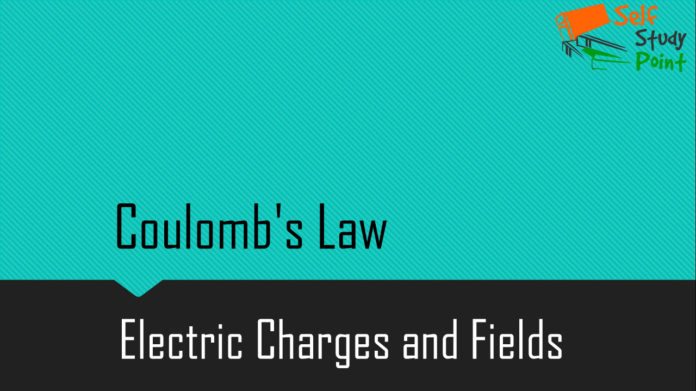Coulomb’s law is a quantitative statement about the force between two point charges.

When the linear size of charged bodies are much smaller than the distance separating them, the size may be ignored and the charged bodies are treated as point charges.

Coulomb measured the force between two point charges and found that it varies inversely as the square of the distance between the charges and was directly proportional to the product of the magnitude of the two charges and acted along the line joining the two charges.

Thus, if two point charges q1, q2 are separated by a distance r in vacuum, the magnitude of the force (F) between them is given bywhere k is a constant of proportionality, called electrostatic force constant. The value of k depends on the nature of the medium between the two charges and the system of units chosen to measure F, q1 ,q2 and r.where ε0 is called permittivity of free space.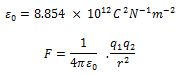### Units of charges:

• The SI unit of charge is coulomb. In the above equation, if q1 = q2 = 1C and r = 1m, then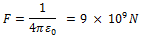• In electrostatic cgs system, the unit of charge is known as electrostatic unit of charge (e.s.u. of charge) or statcoulomb (stat C)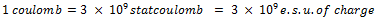• In electromagnetic cgs system, the unit of charge is abcoulomb or electromagnetic unit of charge (e.m.u of charge).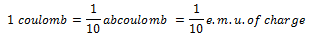## Coulomb’s Law in vector form

1. Let the position vectors of charges q1 and q2 be r1 and r2 respectively [see Fig 1].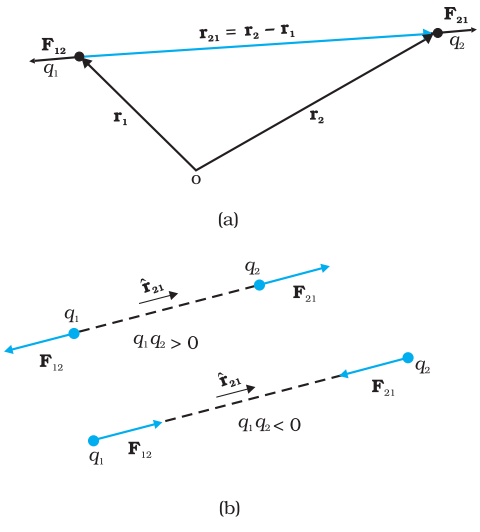Fig. 1 (a) Geometry and (b) Forces between charges

• We denote force on q1 due to q2 by F12 and force on q2 due to q1 by F21.
• The two-point charges q1 and q2 have been numbered 1 and 2 for convenience and the vector leading from 1 to 2 is denoted by r21: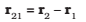In the same way, the vector leading from 2 to 1 is denoted by r12: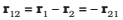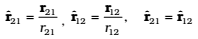Coulomb’s force law between two point charges q1 and q2 located at r1 and r2 is then expressed as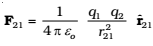The force F12 on charge q1 due to charge q2,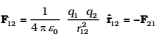Thus, Coulomb’s law agrees with Newton’s third law.